Question

# Use Lagrange multipliers to find the min and max of f(x,y,z) = x2-y2+ 2z subject to the constraint x2 + y2 + z2 = 1.

Use Lagrange multipliers to find the min and max of f(x,y,z) = x2-y2+ 2z subject to the constraint x2 + y2 + z2 = 1.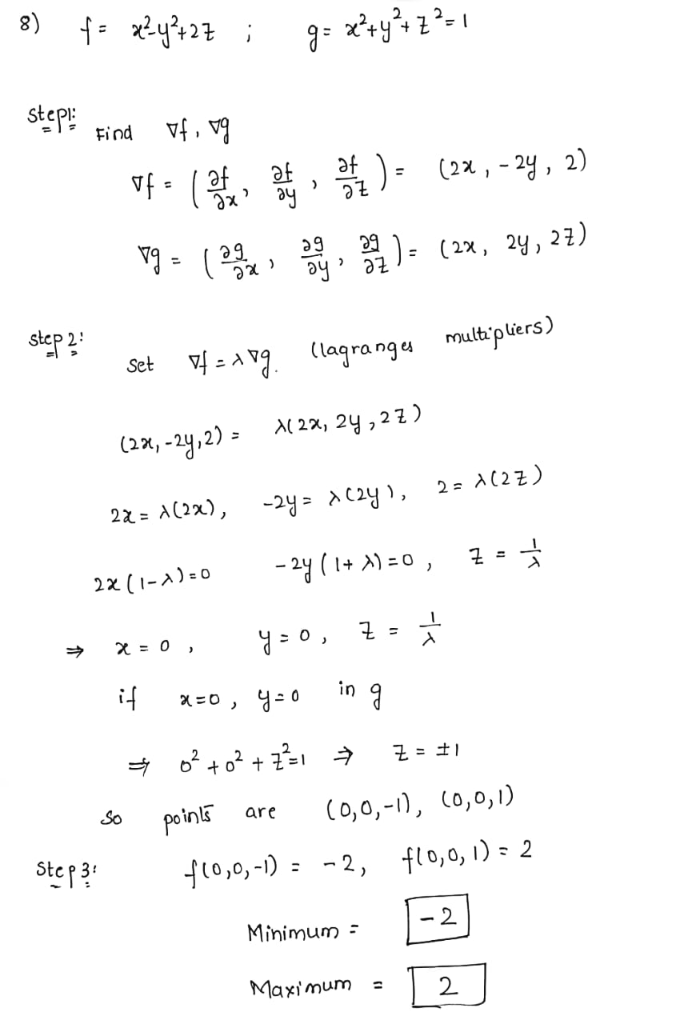#### Earn Coins

Coins can be redeemed for fabulous gifts.

Similar Homework Help Questions
• ### Use Lagrange multipliers to find both the maximum and minimum values of f(z, y, z) = 2x + y-2z on...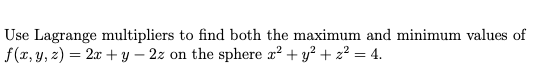Can you help me? This is calculus 3. Use Lagrange multipliers to find both the maximum and minimum values of f(z, y, z) = 2x + y-2z on the sphere r2 + y2 + z2-4. Use Lagrange multipliers to find both the maximum and minimum values of f(z, y, z) = 2x + y-2z on the sphere r2 + y2 + z2-4.

• ### (1 point) Use Lagrange multipliers to find the maximum and minimum values of f(x, y, z)...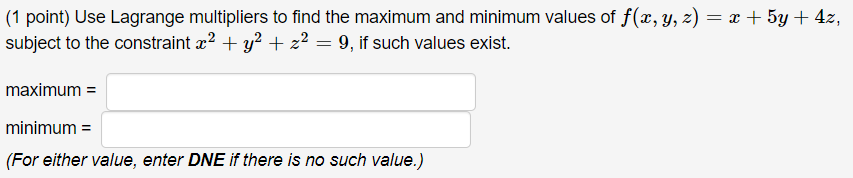(1 point) Use Lagrange multipliers to find the maximum and minimum values of f(x, y, z) = x + 5y + 4z, subject to the constraint x2 + y2 + z2 = 9, if such values exist. maximum = minimum = (For either value, enter DNE if there is no such value.)

• ### Use Lagrange multipliers to find the maximum and minimum values of the function subject to the given constraint. (If a v...

Use Lagrange multipliers to find the maximum and minimum values of the function subject to the given constraint. (If a value does not exist, enter NONE.) f(x,y,z) = x2 + y2 + z2;   x4 + y4 + z4 = 1

• ### use Lagrange Multipliers to find absolute max & min values of the function f(x,y) with constraint...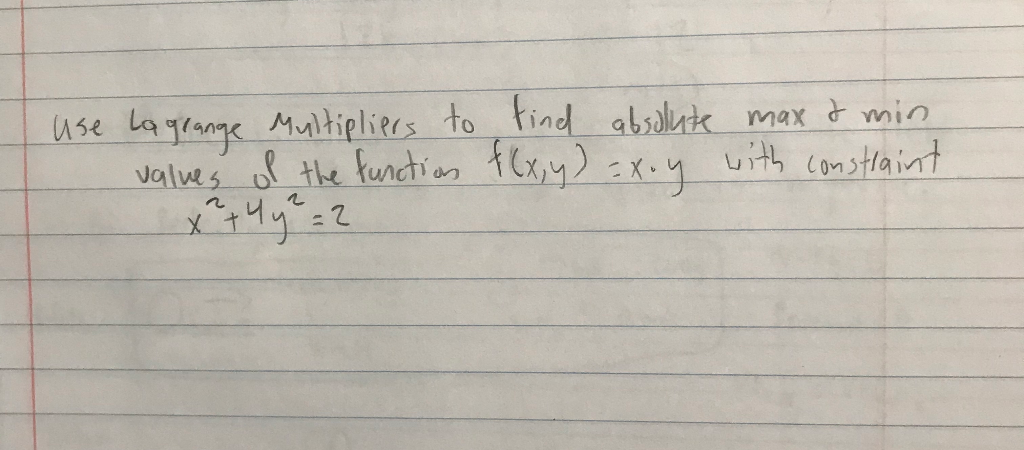use Lagrange Multipliers to find absolute max & min values of the function f(x,y) with constraint X. y 2

• ### Optimize f(x,y,z) = x2+y4+z2 subject to the constraints x3-y2= 1 and z3+x2= 1 Use the second deri...

Optimize f(x,y,z) = x2+y4+z2 subject to the constraints x3-y2= 1 and z3+x2= 1 Use the second derivative test to try to classify the critical point as a maximum or minimum. Explain why the method of Lagrange multipliers is failing for this example. Use the definition of the derivative to classify the extrema.

• ### Use the method of Lagrange multipliers to find the extreme value of the function f(x, y,...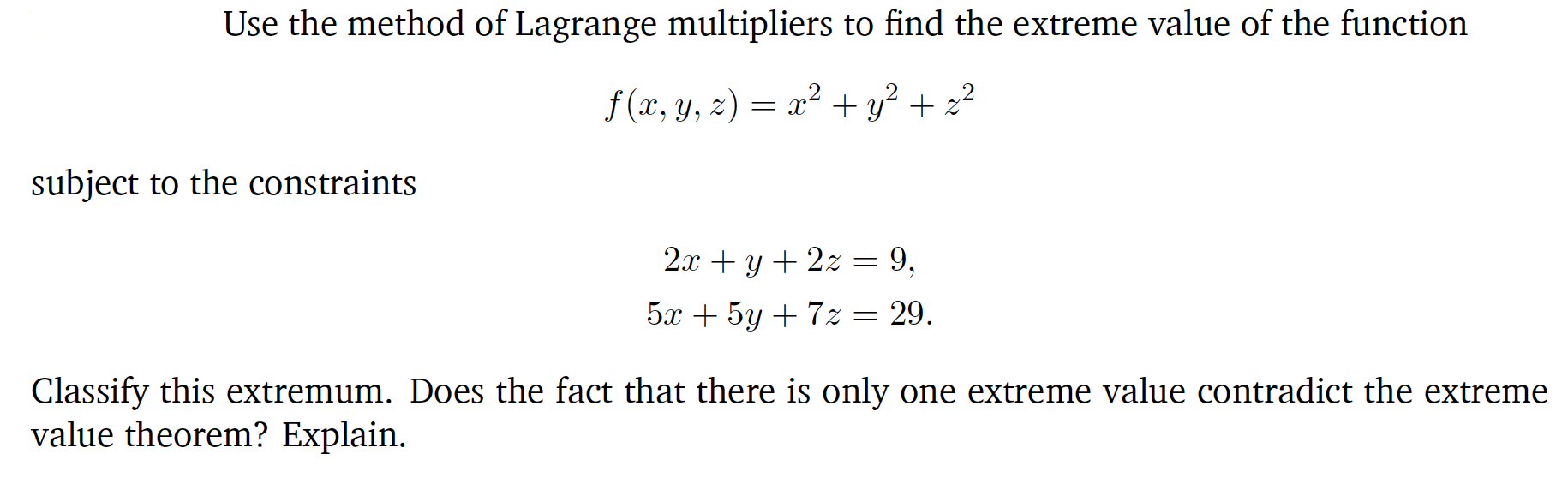Use the method of Lagrange multipliers to find the extreme value of the function f(x, y, z) = x2 + y2 + 22 subject to the constraints 2x + y + 2z = 9, 5x + 5y + 72 = 29. Classify this extremum. Does the fact that there is only one extreme value contradict the extreme value theorem? Explain.

• ### Use Lagrange's Multipliers to find the extreme values of the function f(x, y, z) = 2x...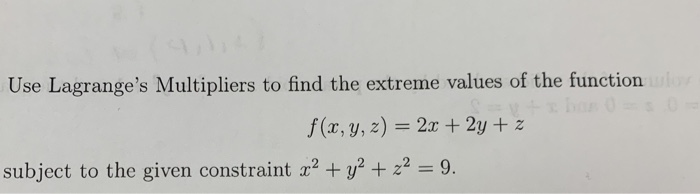Use Lagrange's Multipliers to find the extreme values of the function f(x, y, z) = 2x + 2y + z subject to the given constraint x2 + y2 + z2 = 9.

• ### Use Lagrange multipliers to find the ends of f(x,y)= 2x2 +3y2 subject to the constraint 3x...

Use Lagrange multipliers to find the ends of f(x,y)= 2x2 +3y2 subject to the constraint 3x + 4y = 59

• ### Chapter 15, Review Exercises, Question 017 Use Lagrange multipliers to find the maximum and minimum values...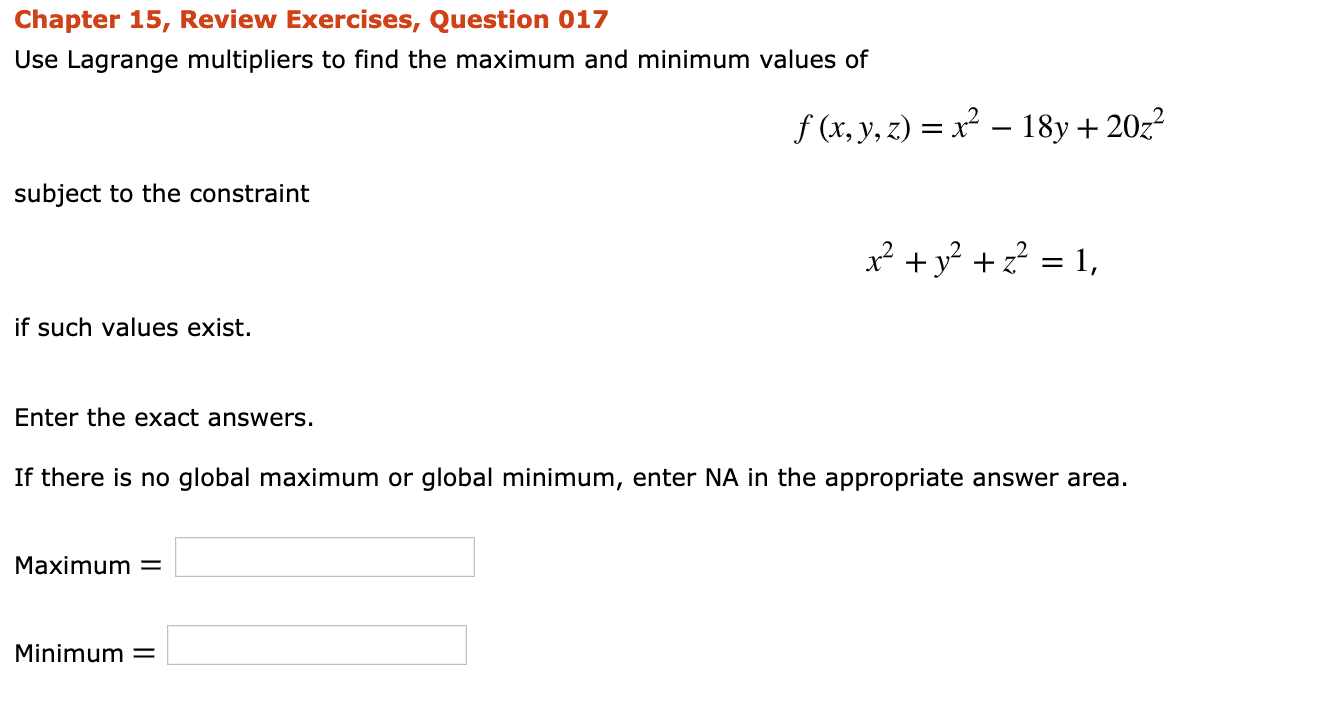Chapter 15, Review Exercises, Question 017 Use Lagrange multipliers to find the maximum and minimum values of f (x, y, z) = x² – 18y+ 2022 subject to the constraint x2 + y2 + z2 = 1, if such values exist. Enter the exact answers. If there is no global maximum or global minimum, enter NA in the appropriate answer area. Maximum = Minimum =

• ### Solve the following problems by USING Lagrange multipliers. (a) Find the maximum and minimum values of f(x, y, z) = x^2...

Solve the following problems by USING Lagrange multipliers. (a) Find the maximum and minimum values of f(x, y, z) = x^2 + y^2 + z^2 subject to the constraint (x − 1)^2 + (y − 2)^2 + (z − 3)^2 = 4 (b) Find the maximum and minimum values of f(x, y, z) = x^2 + y^2 + z^2 subject to the constraints (x − 1)^2 + (y − 2)^2 + (z − 3)^2 = 9 and x − 2z...